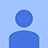# Đề thi Olympic Tiếng Anh lớp 10 vòng 1 năm học 2013 - 2014

1 712

## Đề thi Olympic Tiếng Anh lớp 10

Đề thi Olympic Tiếng Anh lớp 10 vòng 1 năm học 2013 - 2014. Tài liệu gồm 3 đề thi mỗi đề gồm có 10 câu hỏi trắc nghiệm. Hi vọng rằng với tài liệu này các bạn học sinh lớp 10 sẽ đạt được kết quả cao trong kì thi Olympic Tiếng Anh sắp tới.

Đề thi Olympic Tiếng Anh lớp 10 vòng 1 năm học 2012 - 2013

Đề cương ôn thi Tiếng Anh lớp 10 cả năm và bài tập có đáp án

### Đề thi Olympic Tiếng Anh lớp 10 vòng 1 đề thi số 1

Câu 1. Which of the following is a graph of y ≤ 5x?E. None of themCâu 2. Personal computers are given a final inspection after being built. Three types of defects are identified: critical, major and minor. The chart above shows the results of these inspections. Computers with either critical or major defects are deemed unusable and are destroyed. What percentage of computers produced are deemed usable?

A. 89% B. 91% C. 95% D. 98% E. 87%Câu 3. Given that ∠ABC is a right angle, what is the measure of ∠DBE?

A. 50 B. 200 C. 1400 D. 800 E. 500Câu 4. Use the map above to answer the question that follows. If you average 55 miles per hour, about how long will it take to drive from Grady to Minden?

A. 10 to 20 minutes
B. 25 to 35 minutes
C. 40 to 50 minutes
D. More than 55 minutes
E. None of them

Câu 5. Which one of the following objects is most like a geometric plane?

A. Tabletop B. Water glass C. Ice cream cone
D. Shoe box E. None of them

Câu 6. Jake went for a 5-mile run. When he started the run, his watch read 10:05. When he finished the run, it read 10:32. What was his average lap time?

A. 27 minutes per mile B. 5 minutes, 4 seconds per mile
C. 32 minutes per mile D. 5 minutes, 24 seconds per mile
E. None of themCâu 7. What is the MEDIAN height of the five office buildings shown in this list?

A. 157 feet B. About 432 feet C. 440 feet
D. 517 feet E. 368 feet

Câu 8. A king-size water-bed mattress whose dimensions are 7 feet by 6 feet by 6 inches is filled with water. If the weight of a cubic foot of water is 45 pounds, how much will the water in the mattress weigh?

A. 607.5 pounds B. 945 pounds
C. 1,890 pounds D. 11,340 pounds
E. 22,680 pounds

Câu 9. Marie has been hired to paint the walls and ceilings of three rooms. Room 1 is 12 feet by 12 feet, room 2 is 10 feet by 15 feet and room 3 is 10 feet by 12 feet. All three rooms are 10 feet high. If a gallon of paint covers between 250 and 300 square feet, what is the best estimate of the number of whole gallons of paint that Marie needs?

A. 2 B. 5 C. 8 D. 10 E. 12Câu 10. The graph above shows the amount of rustproof paint required to paint metal tanks made by Tanks-R-Us. About how much paint will be required to paint a 1500-gallon tank?

A. 1.5 gallons B. 5 gallons
C. 10 gallons D. 12 gallons
E. None of them

### Đề thi Olympic Tiếng Anh lớp 10 vòng 1 đề thi số 2

Câu 1. Which of these graphs represents a function?E. None of them

Câu 2. If a circular pool has a diameter of 20 feet, what is the area of the pool to the nearest square foot?

A. 31 square feet B. 1256 square feet
C. 63 square feet D. 628 square feet
E. 314 square feetCâu 3. In the house plan shown above, figure FEB is similar to figure FDA. What is the length of segment AD?

A. 12 feet B. 20 feet C. 30 feet D. 35 feet E. 40 feetCâu 4. What is the area of a circle with d = 8x - 6?

A. (16x - 12)π
B. (28x + 9)π
C. (16x2 + 12x + 9)π
D. (16x2 - 24x + 9)π
E. (16x2 + 24x - 9)π

Câu 5. Lou would like to exchange 354 British pounds for U.S. dollars. If 1 U.S. dollar is equal to 0.59 British pounds, how many U.S. dollars will Lou receive?

A. \$145.14 B. \$208.86 C. \$600.00 D. \$863.41 E. \$900.00

y = 3x
2x + y = 15

Câu 6. What is the solution of the system of linear equations above?

A. (0,15) B. (5, 5) C. (15, 45) D. (10, 25) E. (3, 9)Câu 7. The average high temperatures for a city during the month of October are shown in the table above. What is the mean of the average high temperatures?

A. 760 B. 790 C. 820 D. 880 E. 890

Câu 8. What is the equation of the line passing through the points (1, 2) and (3, 4)?

A. y = x + 1 B. y = x - 1 C. x + y = 1
D. x + y = 2 E. x + y = 3

Câu 9. The measure of an angle in degrees is 3x. Which of these represents the measure of its supplement?

A. 3x + 90 B. 3x + 180 C. 90 - 3x D. 180 - 3x E. 180 + 3xCâu 10. Peter uses a 12-foot ladder to wash windows at his house. What is the distance (x) from the base of the wall to the bottom of the ladder?

A. 4√5 B. 4√13 C. 16√5 D. 16√13 E. 8√13

Đánh giá bài viết
1 712
0 Bình luận
Sắp xếp theo